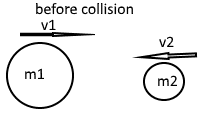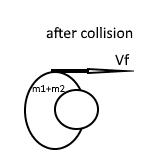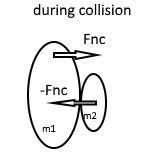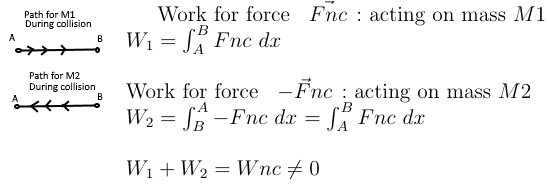# Inelastic collision and the sum of internal forces

## Homework Statement

Suppose I have a system which contains two bodies m1 and m2 with initial velocities v1 and v2 , respecitvely.
they hurl toward each other and make an inelastic collision. such that they are now one body of mass
m1 + m2I know that the difference in momentum is conserved before collision and after collision, so that: ΔP = 0

but during that time of collision , non-conservative force Fnc act on both bodies and thus the difference in mechanical energy before the collision and after the collision is not conserved ,so that : Eb - Ea = Wnc
Eb = mechanical energy after collision
Ea = mechanical energy before collision
Wnc = Work done by non conservative force

However , I have a problem at understanding why the energy is not conserved , If during the time of collision, both bodies have a non-conservative force ' Fnc ' acting upon them , and this force is equal in magnitude but opposite in direction on each body ,then how can it be that these two forces do not cancel out each other ( the sum of internal forces equals to zero ) so that the total work done by the sum of non-conservative forces is zero? I say that Wnc = 0 since I suppose that Wnc = ∫( -Fnc + Fnc)dr = 0 ( the forces and dr are vectors , and the integral is path integral )

So since Wnc = 0 by the sum of internal forces , then the diffrence in mechanical energy should be conserved so that Eb - Ea = 0 , but this is not the case , why?Eb - Ea = Wnc

-

## Answers and Replies

haruspex
Science Advisor
Homework Helper
Gold Member
2020 Award
then how can it be that these two forces do not cancel out each other
The work done on one is ##\int \vec F.\vec{dr}##. For the other, using common mass centre as origin, both force and displacement vectors change sign, so the work done is the same sign for each, no cancellation.

The work done on one is ##\int \vec F.\vec{dr}##. For the other, using common mass centre as origin, both force and displacement vectors change sign, so the work done is the same sign for each, no cancellation.
The way I thought about is, that for the sake of simplicity , the non conservative force displaces on the x axis , so the work done by the non conservative forces 'Fnc' on each mass:So from what I did in the sketch above I got that the total work done by the non-conservative forces Wnc is not zero , do you think this is correct?

haruspex
Science Advisor
Homework Helper
Gold Member
2020 Award
The way I thought about is, that for the sake of simplicity , the non conservative force displaces on the x axis , so the work done by the non conservative forces 'Fnc' on each mass:
View attachment 211301
So from what I did in the sketch above I got that the total work done by the non-conservative forces Wnc is not zero , do you think this is correct?
That's the idea, but of course the A and B are not the same between your two pictures.

That's the idea, but of course the A and B are not the same between your two pictures.
Ok , but if I now suppose that the collision is elastic , then how will Wnc = 0 ? ( if the collision is elastic then Fnc is conservative force, but still , Wnc is not 0 according to the derivation in the last image )

Bump

haruspex
Science Advisor
Homework Helper
Gold Member
2020 Award
Ok , but if I now suppose that the collision is elastic , then how will Wnc = 0 ? ( if the collision is elastic then Fnc is conservative force, but still , Wnc is not 0 according to the derivation in the last image )
I found your diagrams in post #3 a bit hard to interpret since, as I posted, the A and B in one cannot be the same as the A and B in the other.
It would be clearer to consider both approaching their common mass centre, C say.
By conservation of momentum, there will be an instant when each is stationary relative to the uniform motion of their common mass centre. If they now stick together, work has been lost, but if they spring apart again then some or all will be recovered. Only then do you discover whether the forces were conservative.